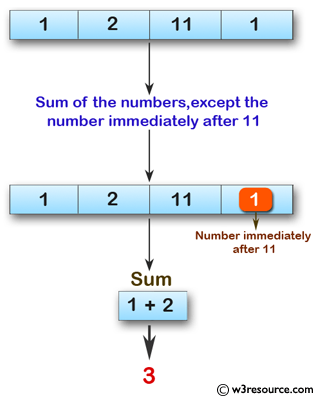﻿ Swift Array Programming Exercise: Compute the sum of the numbers of a given array of integers except the number immediately after a 11 - w3resource# Swift Array Programming Exercises: Compute the sum of the numbers of a given array of integers except the number immediately after a 11

## Swift Array Programming: Exercise-26 with Solution

Write a Swift program to compute the sum of the numbers of a given array of integers except the number immediately after a 11.

Pictorial Presentation:Sample Solution:

Swift Code:

``````func sum_11(array_nums: [Int]) -> Int {
var sum = 0
var ans = true
for x in 0..<array_nums.count
{
if array_nums[x] != 11 && ans == true {
sum += array_nums[x]
ans = true

}
else
{
ans = false
}

}

return sum
}

print(sum_11(array_nums: [1, 2, 11, 1]))
print(sum_11(array_nums: [1, 1]))
print(sum_11(array_nums: [1, 2, 2, 1, 11, 5]))
print(sum_11(array_nums: [1, 2, 2, 1, 11]))
```
```

Sample Output:

```3
2
6
6
```

Swift Programming Code Editor:

Improve this sample solution and post your code through Disqus

What is the difficulty level of this exercise?

﻿